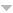# Library

Author TitleSalmon G. A treatise on analitic geometry of three dimensions
Coolidge J.L. A treatise on algebraic plane curves.
Schatten R. A Theory of Cross-Spaces
Brenkek William Charles A text-book on Advanced Algebra and Trigonometry with Tables
Ferrar W.L. A text-book of convergence.
Birkhoff Garrett, MacLane Saunders A survey of modern algebra
Bruck Richard Hubert A Survey of Binary Systems. 3-rd print.correct. B.20
Wold H. A study in the analysis of stationary time series
NULL A spectrum of Mathematics. Essays Presented to H.G.Forder.
Rainville E.D. A short course in differential equations.
Ball Walter W. Rouse A short account of the history of mathematics
Karlin Samuel, Taylor Howard M. A Second Course in Stochastic Processes
Bopp Nicole A Propos des Distributions de Type Positif Pour les Fonctions Invariantes par un Groupe Fini D'isometries. These
de Boor C. A Practical Guide to Splines
Wojtaszczyk P. A Mathematical Introduction to Wavelets
Baker H.F. A locus with 25920 selftransformations
Coolidge J.L. A history of geometrical methods.
Yates R.C. A handbook on curves and their properties
Renaut J. A groupoid approach to C*-algebras.
Edwards R.E. A Formal Background to Mathematics. 2b. A Critical Approach to Elementary Analysis.
Edwards R.E. A Formal Background to Mathematics. 2a. A Critical Approach to Elementary Analysis.
Karlin Samuel, Taylor Howard M. A First Course in Stochastic Processes. (Second edition)
Karlin S. A First Course In Stochastic Processes
Ralston A. A First Course In Numerical Analysis
Mustonen V. A Duality Theorem on l^p-spaces.
Hardy G.H. A course of pure mathematics.
Whittaker E.T., Watson G.N. A Course of Modern Analysis. An Introduction to the General Theory of Infinite Processes and of Analytic Functions; With an Account of the Principal Transcendental Functions
Narayan Sh. A course of mathematical analysis.
Rubinstein Z. A course in ordinary and partial differential equations.
Kendall M.G. A Course in Multivariate Analysis
Conway J.B. A course in functional analysis.
Gierz G., Hofmann K.H., Keimel K., Lawson J.D., Mislove M., Scott D.S. A Compendium of Continuous Lattices.
Julia G. 2 pte.Lecons redigees par J.Dufresnoy
Julia G. 2 pte
Julia G. 1 pte. Lecons redigees par R. Marrot
Julia G. 1 pte Receuillies et redigees par M.Brelton et Rene de Possel
Julia G. V. 1. Redige aves la coloboration de Pierre Boos, Rene Harmegnies et Roger Julia.
Julia G. Fasc. 5. Geometrique infinitesimale. 2me pte
Julia G. Fasc. 4. Cinematique et geometrie cinematique
Julia G. Fasc. 3. Geometrique infinitesimale. 1me pte